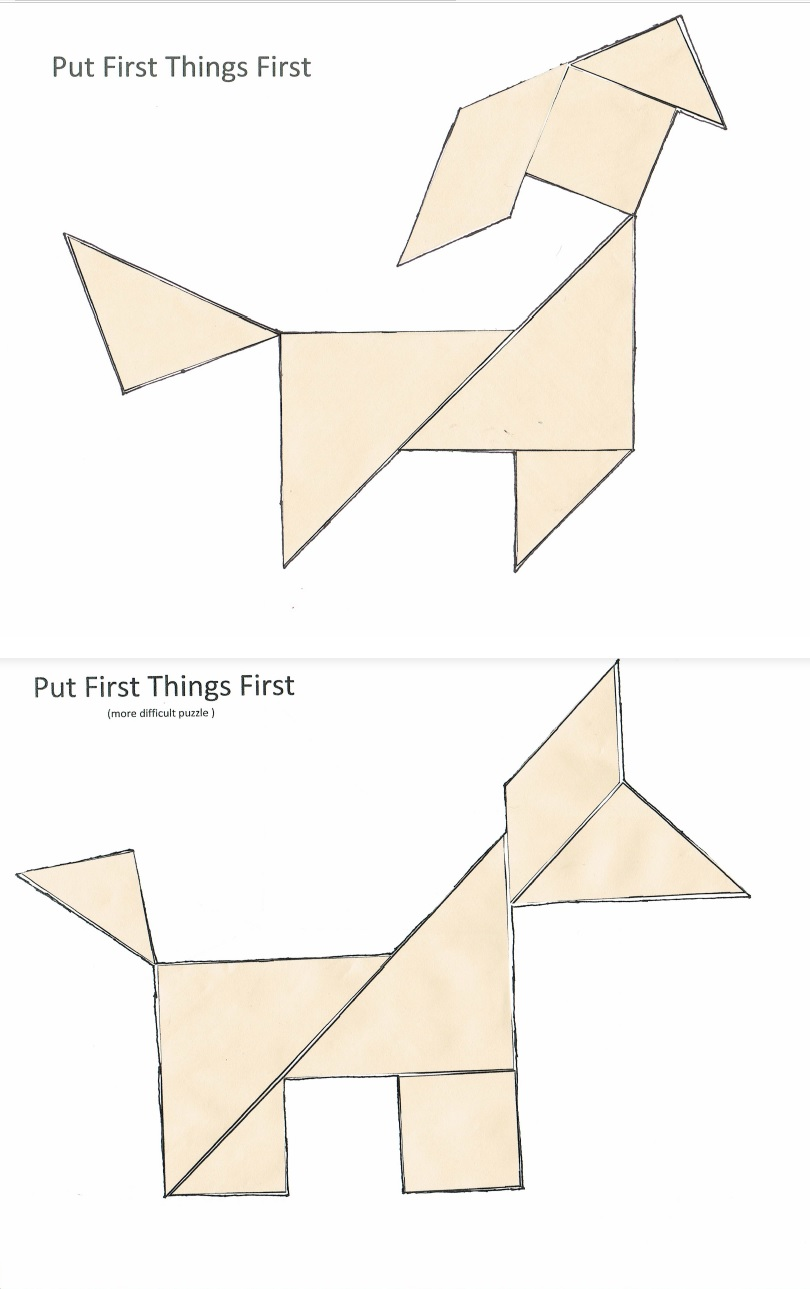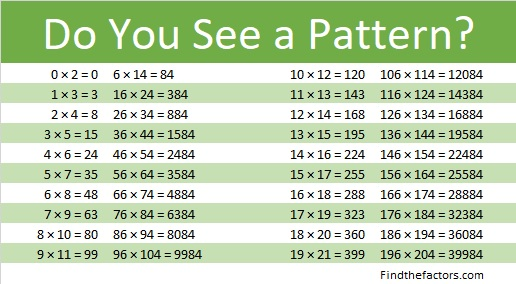# 1269 Tangram Witch Puzzle

If you have the seven tangram pieces, then you can create this Halloween witch riding across the moon (or a paper plate). Best Witches creating all kinds of things with those fabulous tiles!In case you would like to know some facts about the number 1269, here’s what I’ve learned:

• 1269 is a composite number.
• Prime factorization: 1269 = 3 × 3 × 3 × 47, which can be written 1269 = 3³ × 47
• The exponents in the prime factorization are 3 and 1. Adding one to each and multiplying we get (3 + 1)(1 + 1) = 4 × 2 = 8. Therefore 1269 has exactly 8 factors.
• Factors of 1269: 1, 3, 9, 27, 47, 141, 423, 1269
• Factor pairs: 1269 = 1 × 1269, 3 × 423, 9 × 141, or 27 × 47
• Taking the factor pair with the largest square number factor, we get √1269 = (√9)(√141) = 3√141 ≈ 35.623031269 is the difference of two squares four different ways:
37² – 10² = 1269
75² – 66² = 1269
213² – 210² = 1269
635² – 634² = 1269

# 884 Put First Things First

Tangrams have two large pieces, three medium size pieces, and two small pieces.Most Tangram puzzles are easier to solve if you figure out where to put the two big triangles first. Making a daily plan is easier if you figure out where to schedule the important items like homework and chores first. One of the Seven Habits of Highly Effective People is Put First Things First.

I wrote a 30-40 minute lesson plan to teach habit number 3 of the seven habits with the seven Tangram shapes. The lesson has now been taught to a third of a local elementary school. It was taught to students from first grade to sixth, and all of them really liked the lesson. The teachers who taught it enjoyed it very much as well. The rest of the school will be taught the same lesson later.

I made a pdf copy of the lesson plan here: Put First Things First. You can use it if you would like to teach that principle to your children or your students.  Part of the lesson is reading the adorable book, A Small Brown Dog with a Wet Pink Nose, by Stephanie Stuve-Bodeen. Our county library system had more than enough copies for us to use. It is also available on Amazon.com.The Tangram square above was copied on light brown paper so each student in the class could make their own small brown dog.Students could make both dogs or just one of them. Ability levels vary in surprising ways. There were a few first graders who could put the Tangram puzzle together without any help while a few of the older kids struggled. It was okay if a student had difficulty putting the puzzle together. In fact, I made that potential difficulty an important part of the lesson plan. After playing with the puzzle pieces, some students chose to glue the pieces onto the puzzle. Some of them used crayons to add details to their dogs.

The book and the puzzle were the funnest parts of the lesson, but the lesson began with a serious discussion. We used a document camera to show the four time quadrants to the classes.(I used the term “Pressing” instead of the more common term “Urgent.” Elementary students probably don’t know what either of those words mean, but they do know what “Pressure” means. They will feel a lot of pressure if they wait until the last minute to do something important. They might feel peer pressure to follow the crowd. Pressing and pressure have the same root word.)

So go ahead, click on the pdf file, Put First Things First, and teach planning and responsibility using the seven Tangram shapes.

Now I’ll write a little bit about the number 884:

884 is the hypotenuse of four Pythagorean triples.

• 84-880-884 which is 4 times (21-220-221)
• 340-816-884 which is (5-12-13) times 68
• 416-780-884 which is (8-15-17) times 52
• 560-684-884 which is 4 times (140-171-221)

If we had more than ten fingers, 884 might be written in some of these interesting ways:

• 2D2 in BASE 18 (D is 13 base 10), because 2(18²) + 13(18) + 2(1) = 884
• 202 in BASE 21, because 2(21²) + 2(1) = 2(441 + 1) = 2(442) = 884
• QQ in BASE 33 (Q is 26 base 10), because 26(33) + 26(1) = 26(34) = 884
• Q0 in BASE 34 because 26(34) = 884

What are the factors of 884?

• 884 is a composite number.
• Prime factorization: 884 = 2 × 2 × 3 × 73, which can be written 884 = 2² × 13 × 17
• The exponents in the prime factorization are 2, 1, and 1. Adding one to each and multiplying we get (2 + 1)(1 + 1)(1 + 1) = 3 × 2 × 2 = 12. Therefore 884 has exactly 12 factors.
• Factors of 884: 1, 2, 4, 13, 17, 26, 34, 52, 68, 221, 442, 884
• Factor pairs: 884 = 1 × 884, 2 × 442, 4 × 221, 13 × 68, 17 × 52, or 26 × 34,
• Taking the factor pair with the largest square number factor, we get √884 = (√4)(√221) = 2√221 ≈ 29.732137884 is in this cool pattern: